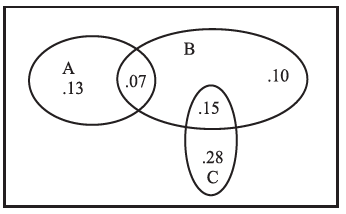The accompanying Venn diagram shows three events, A, B, and C, and also the probabilities of the various intersections (for instance, P (A ∩ B) = .07. Determine
(a) P (A ∪ B)
(b) P (A ∩ B)
The accompanying Venn diagram shows three events, A, B, and C, and also the probabilities of the various intersections (for instance, P (A ∩ B) = .07. Determine
(a) P (A ∪ B)
(b) P (A ∩ B)Solution:

It is given thatFrom the Venn Diagram given

(a)( b)